```

```
Derivation of the 32 Crystal Classes   (Part Three)
```

```

back to homepage

```
```
In Part One we started the derivation of the first 27 Crystal Classes (out of the total of 32 Classes). These Classes, as represented by the stereographic projections of their symmetry elements and of the face poles of their most general Forms, are given in a table, presented in Part One.
In Part Two we succeeded in deriving all these 27 Classes, and started to check whether or not still other symmetry combinations beyond these 27, but still having at most one more-than-2-fold axis, are generated when we add either a center of symmetry, or a 2-fold rotation axis perpendicular to the n-fold axis, or a mirror plane parallel to that n-fold axis, or a mirror plane perpendicular to that axis to the symmetry contents of the new found Classes.
We started to do that with the Classes of column D. With respect to that column we already added a center of symmetry to the symmetry content of its Classes. No new Classes were found.
In the present Part we will continue this check. A center of symmetry will be added to the Classes in the columns E, F and G. When this is done we have checked the (effect of the) addition of a center of symmetry to the (symmetry content of the) Classes of columns D, E, F and G. Then a 2-fold rotation axis will be added to the Classes of the columns D, E, F and G. The same will be done with respect to the other two mentioned symmetry elements.

Adding a center of symmetry to (the Class represented by) E2 (= the first Class of column E) yields G2. See Figure 1.Figure 1. E2---G2 Addition of a center of symmetry generates four new motifs, and implies a horizontal mirror plane, and two more 2-fold rotation axes.

Let's explain this by invoking real motifs (instead of just calling the face poles motifs).
See Figure 1a.Figure 1a.
Stereogram of E2 with real motifs (represented by comma's).
Addition of a center of symmetry means that each motif sends an inverted copy to the opposite location. The inversion of the motifs
a, b, c, d is indicated to the right of the stereogram of E2 :
The upper motif
a sends its inverted image to a location right below the upper motif d, resulting in motif pair, the members of which are symmetric with respect to the plane of projection.
The upper motif
b sends its inverted image to a location right below the upper motif c, resulting in motif pair, the members of which are symmetric with respect to the plane of projection.
The upper motif
c sends its inverted image to a location right below the upper motif b, resulting in motif pair, the members of which are symmetric with respect to the plane of projection.
The upper motif
d sends its inverted image to a location right below the upper motif a, resulting in motif pair, the members of which are symmetric with respect to the plane of projection.
The resulting pattern of real motifs is depicted in the lower left image of this Figure.
This pattern now implies a horizontal mirror plane.
Where two mirror planes perpendicular to each other intersect, a 2-fold rotation axis is implied (See Figure 1b).
The resulting symmetry configuration is that of G2.

```
```Figure 1b.
If we have two mirror planes (m) intersecting at right angles, then a 2-fold rotation axis coinciding with the line of intersection is implied : Motif a maps onto motif b when it is rotated by 1800 about the line of intersection. Likewise motif a' maps onto motif b' when thus rotated. The implied 2-fold rotation axis is perpendicular to the plane of the drawing, and is indicated by a small blue ellipse.

```

```
Adding a center of symmetry to (the Class represented by) E3 (= the second Class of column E) yields E3, because E3 already has a center of symmetry which is evident by the distribution of motifs. See Figure 2.Figure 2. E3---E3 Addition of a center of symmetry generates no new motifs, because E3 already has a center of symmetry.

```
```
Adding a center of symmetry to E4 yields G4.
See Figure 3 ( The rotation, as seen in the drawings, of the motif pattern is immaterial).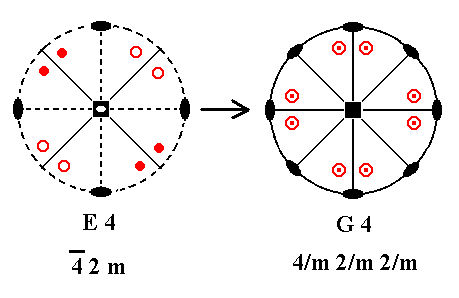Figure 3.   E4---G4. Addition of a center of symmetry generates eight new motifs, and implies a horizontal mirror plane, and two more vertical mirror planes, and two more 2-fold rotation axes. Because the new motif pattern will be mapped onto itself every 900 the n-fold axis, which originally was a 4-fold roto-inversion axis, becomes a 4-fold rotation axis.

```
```
Adding a center of symmetry to E5 yields G5. See Figure 4.Figure 4. E5---G5. Addition of a center of symmetry generates twelve new motifs, implies three more vertical mirror planes and three more 2-fold rotation axes. Because the new motif pattern maps onto itself every 600 the n-fold axis, which was originally a 6-fold roto-inversion axis, becomes a 6-fold rotation axis.

```
```
Summing up : Adding a center of symmetry to the Classes of column E yielded G2, E3, G4 and G5. So nothing new is generated.
```
```
Now we will add a center of symmetry to the Classes of column F :
Addition of a center of symmetry to (the Class represented by) F3 (= the first Class of column F) yields E3. See Figure 5.Figure 5. F3---E3. Addition of a center of symmetry generates six more motifs and implies three 2-fold rotation axes bisecting the angles between the three vertical mirror planes. The new motif configuration is such that the n-fold axis, which was originally a 3-fold rotation axis, has become a 3-fold roto-inversion axis, because if we rotate the new motif pattern by 1200 about that axis immediately followed by an inversion in a point on that axis, then the pattern will be mapped onto itself.

```
```
Addition of a center of symmetry to F4 yields G4. See Figure 6.Figure 6. F4---G4. Addition of a center of symmetry generates eight new motifs and implies four 2-fold rotation axes.

```
```
Addition of a center of symmetry to F5 yields G5. See Figure 7.Figure 7. F5---G5. Addition of a center of symmetry generates twelve new motifs and implies a horizontal mirror plane and six 2-fold rotation axes.

```
```
Summing up : Adding a center of symmetry to the Classes of column F has yielded E3, G4 and G5. So no new Classes are generated.
```
```
Now we will add a center of symmetry to the Classes of column G :
Addition of a center of symmetry to G2 (= the first Class of column G) yields G2, because G2 already has a center of symmetry. See Figure 8.Figure 8. G2---G2

```
```
Addition of a center of symmetry to G4 (= the second Class of column G) yields G4, because G4 already has a center of symmetry. See Figure 9.Figure 9. G4---G4

```
```
Addition of a center of symmetry to G5 yields G5, because G5 already has a center of symmetry. See Figure 10.Figure 10. G5---G5

```
```
Summarizing : Addition of a center of symmetry to the Classes of column G has yielded G2, G4 and G5. So nothing new is generated.
```
```
Next we will look to the effect of the addition of a 2-fold rotation axis perpendicular to the n-fold axis to the (symmetry content of the) Classes of columns D, E, F and G.
Addition of a 2-fold rotation axis perpendicular to the n-fold axis of the Class represented by D2 (= the first Class of column D) yields D4, or D5, depending on the angle which the added axis makes with the other horizontal 2-fold axes that were already present. We can choose between the possible angles : 600, 300, 450 and 900, as they are permitted in crystals insofar as their rotational symmetry is determined by such angles (In considering the angles between two rotational axes we always choose to deal with the smaller one of the two possible angles). At 900 we already have a 2-fold rotation axis in place.
When the added axis lies exactly between the other two horizontal 2-fold axes, i.e. making an angle of 450 with them (referring here to Class D2), these original axes will not be multiplied because they are mapped onto each other by the added axis. We will finally end up with four horizontal 2-fold axes leading to a 4-fold rotational symmetry of the (vertical) n-fold axis (i.e. n becomes 4) : The Class D4 will then be generated (See Figure 11c).
When its angle is 300 we will end up with six horizontal 2-fold axes leading to a 6-fold symmetry of the (vertical) n-fold axis (i.e. n becomes 6) : The Class D5 will then be generated (See Figure 11d).
When that angle is 600 we'll also end up with six horizontal 2-fold axes leading to a 6-fold rotational symmetry of the n-fold axis, and so also resulting in Class D5 (Figure 11d).
If instead we would add such a 2-fold rotation axis not making an angle of either 300, or 450, or 600 with the existing 2-fold axes, then we would cause the 2-fold axes to proliferate beyond the number permitted for crystals, permitted, in virtue of their periodic internal order (The proof of the permitted rotational symmetries in crystals is given in Part One). The n-fold axis would become such that n is different from its permitted values in crystals.
See Figure 11a, b and c.

We begin with adding a 2-fold rotation axis (perpendicular to the (vertical) n-fold axis) making an angle of 300 with the already existing axis aa (in Figure 11a).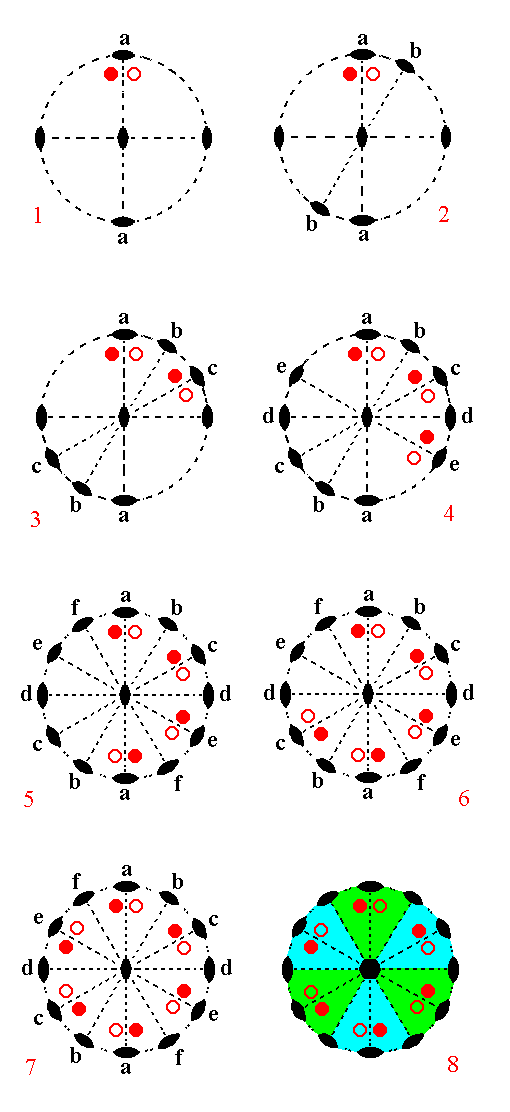Figure 11a.
(1). Two horizontal 2-fold rotation axes initially present.
(Although they do imply a third 2-fold rotation axis perpendicular to both of them, for the moment we will only focus on the horizontal 2-fold axes (lying in the plane of the drawings), and to begin with we focus only on axis aa).
An upper motif (small solid red circle) with a general position is duplicated by the axis
aa as indicated, resulting in a motif pair consisting of one upper and one lower motif.
(2). Addition of a 2-fold rotation axis
bb (perpendicular to the n-fold axis) making an angle of 300 with axis aa.
(3). The axis
aa is duplicated by axis bb and so are the motifs as indicated, resulting
in axis
cc.
(4). The axis
dd (which was already present) duplicates cc and its associated (as drawn) motifs, resulting in axis ee
(5). The axis
ee duplicates axis dd resulting in axis ff which duplicates the motifs (drawn) associated with ee. The resulting motif pair becomes associated with the lower end ( lower in the drawing) of axis aa.
(6). The axis
bb duplicates this motif pair again as indicated.
(7). The axis
dd duplicates the resulting motif pair again.
(8). The resulting motif pattern turns out to be consistent with
six-fold rotational symmetry of the n-fold axis (i.e. the axis perpendicular to the plane of the drawing).
For an explanation why this is so, see the
Remark directly below.

Remark (Inframe, including one Figure) :

When we add a 2-fold rotation axis (perpendicular to the n-fold axis) which makes an angle of 600 with axis aa, we will also end up with a 6-fold symmetry of the vertical axis (= the n-fold axis perpendicular to the plane of the drawing), see again Figure 11a :

The added 2-fold rotation axis will then be axis cc. Axis dd (already present) will be duplicated by axis cc yielding axis bb. This axis bb will duplicate the motif pair associated with aa resulting in our seeing such a pair at axis cc.
Axis dd duplicates cc yielding axis ee. It also duplicates the motif pair associated with axis cc resulting in a further motif pair associated with axis ee. This axis ee in turn duplicates axis dd yielding axis ff. This axis ff duplicates the motif pair associated with axis ee resulting in a motif pair associated with the lower part (lower as drawn in the Figure) of axis aa. Now all the six horizontal 2-fold rotation axes, aa, bb, cc, dd, ee and ff are in place.
Axis bb duplicates the motif pair associated with the lower part of axis aa resulting in such a pair at the left end of axis cc. Axis dd, finally, duplicates this pair, resulting in a motif pair at the left end of axis ee.
The resulting motif pattern is the same as the one we had obtained for the added 2-fold axis making an angle of 300 with axis aa, so also in this case a 6-fold rotational symmetry results for the n-fold axis (= the axis perpendicular to the plane of the drawings).

```
```
The next Figure shows that when a 2-fold rotation axis (perpendicular to the n-fold axis) making an angle of 1/2(450) (= twenty-two and a half degrees) with the axis aa (as denoted in Figure 11a), is added, an 8-fold symmetry results, an impossibility in crystals.Figure 11b.
(1). Stereogram of the symmetry elements and the face poles of the most general Form of the Class 222, represented by the entry D2 of our table (of the first 27 Crystal Classes to be derived).
(2). Addition of a 2-fold rotation axis perpendicular to the n-fold axis and making an angle of 1/2(450) with the horizontal 2-fold axis (axis
aa in Figure 11a) already present. The added axis is duplicated by the latter 2-fold axis. In compliance with these new 2-fold axes new motifs are generated.
(3). The new axes in their turn duplicate the original axes : The implied axis generates another axis (red) by duplicating the original one. The resulting axis then duplicates the implied axis. The resulting axis (blue) is duplicated by the existing axis
dd (as denoted in Figure 11a) resulting in yet another axis. The axis dd is duplicated by that new axis resulting in yet another axis, which is again duplicated resulting in an eighth axis. So now we have a total of eight horizontal 2-fold rotation axes.
The resulting pattern of horizontal 2-fold rotation axes and the new motif pattern are consistent with 8-fold rotational symmetry which is not possible in crystals because of their periodic internal order.

The above Figure (11b) shows that if a 2-fold rotation axis is added such that it makes a 1/2(450) angle with one or the other horizontal 2-fold axis already present (as in Class D2), then, in multiplying the already existing axes, this results in a rotational symmetry higher than 6, which -- as has been proved -- cannot occur in crystals. Here we end up with an eight-fold rotational symmetry.
When we want to add a 2-fold rotation axis perpendicular to the n-fold axis, it should make an angle of either 450, or 300, or 600 with an already existing 2-fold rotation axis.

So we will first add a 2-fold rotation axis to the Class represented by D2, making a 450 angle with the other axes. Then another 2-fold axis is automatically implied (in compliance with the original (horizontal) 2-fold rotation axes). The motif configuration consistent with these axes has then become such that the n-fold axis has become a 4-fold rotation axis. In this way the Class represented by D4 is derived from D2. See Figure 11c.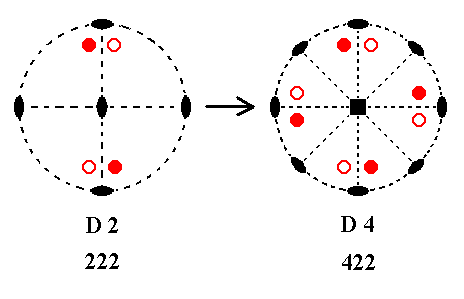Figure 11c. D2---D4. Addition of a 2-fold rotation axis perpendicular to the n-fold axis (and lying exactly midway between the other two horizontal 2-fold axes) generates four new motifs, and implies one more 2-fold rotation axis, because the newly added axis is duplicated by an existing 2-fold axis. The new motif configuration becomes such that the n-fold axis, which was originally a 2-fold rotation axis (perpendicular to the plane of the drawing), becomes a 4-fold rotation axis. The new motif pattern will be mapped onto itself with every rotation by 900 about the n-fold axis (perpendicular to the drawing).

```
```
When we add a 2-fold rotation axis perpendicular to the n-fold axis and making an angle of either 300 or 600 with the existing (horizontal) 2-fold axes, then we will end up with the Class represented by D5. See Figure 11d.Figure 11d. D2---D5. Addition of a 2-fold rotation axis perpendicular to the n-fold axis and making an angle of 300 or 600 with an existing 2-fold rotation axis generates eight new motifs, and implies four more horizontal 2-fold rotation axes, while the n-fold axis, which was originally a 2-fold axis (perpendicular to the plane of the drawing), has become a 6-fold rotation axis (See also (8) of Figure 11a).

```
```
Addition of a 2-fold rotation axis perpendicular to the n-fold axis of D3 yields D5.
See Figure 12.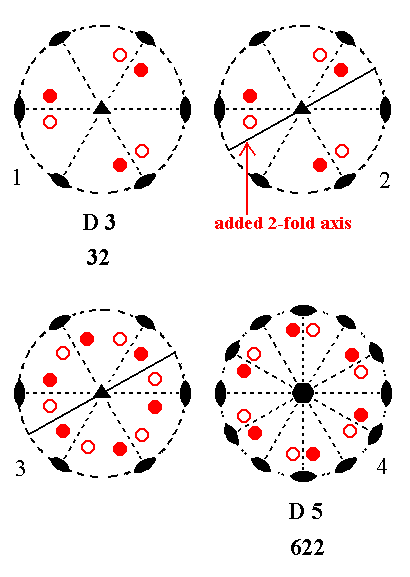Figure 12. D3---D5.
(1). Stereogram of the symmetry elements and of the face poles of the most general Form of Class 32 represented by the entry D3 of our table.
(2). Addition of a 2-fold rotation axis perpendicular to the n-fold axis and bisecting the angle between two already existing 2-fold axes. It accordingly makes an angle of 300 with those axes, but at the same time it makes an angle of 900 with another already existing horizontal 2-fold rotation axis. After the new motif pattern is generated by these axes, other 2-fold rotation axes are then implied. The initially added axis makes an angle of 600 with them. So we -- in one stroke -- have tried out all the permitted angles that the added axis can make with existing axes (In the present case an angle of 450 implies an angle of 150 leading to a forbidden symmetry).
(3). The added 2-fold rotation axis is consistent with the extended pattern of motifs as indicated.
(4). The generated pattern is consistent with
622 symmetry and is represented by the entry D5 of our table.

```
```
Addition of a 2-fold rotation axis perpendicular to the n-fold axis of D4 yields D4.
See Figure 13.Figure 13. D4---D4.
(1). Stereogram of the symmetry elements and of the face poles of the most general Form of Class 422 represented by the entry D4 of our table.
(2). Addition of a 2-fold rotation axis perpendicular to the n-fold axis and making angles of 300 and 600 with existing 2-fold axes implies still more 2-fold axes, such that an 8-fold symmetry will be the result (See also Figure 11b), which is impossible in crystals. So in this case the only angles such an added axis can make with existing axes are 450 and 900. But such axes are already present. Consequently the symmetry content will not be affected by the addition of a 2-fold rotation axis perpendicular to the n-fold axis.

```
```
Addition of a 2-fold rotation axis perpendicular to the n-fold axis of D5 yields D5.
See Figure 14.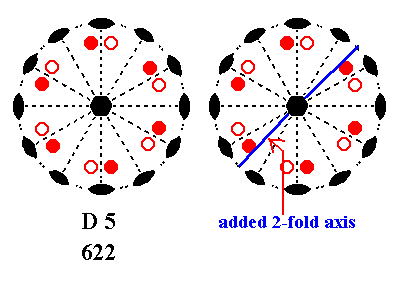Figure 14. Stereogram of the symmetry elements and of the face poles of the most general Form of the Class 622 represented by the entry D5 of our table. When we would add a 2-fold rotation axis making an angle of 450 with one of the existing horizontal 2-fold axes, then -- as can be seen in the right image of the Figure -- an angle (between 2-fold axes) of 150 is implied, which is not permissible in crystals.
Angles of 300, 600 and 900 between existing horizontal 2-fold axes are already present in Class D5, so an addition of a 2-fold rotation axis perpendicular to the n-fold axis of D5 does not affect its symmetry content.

```
```
Summarizing : Addition of a 2-fold rotation axis perpendicular to the n-fold axes of the Classes of column D yielded : D4 and D5, so nothing new is generated.
```
```
Now we will add a 2-fold rotation axis perpendicular to the n-fold axis (the vertical axis) of the Classes of column E.
Addition of a 2-fold rotation axis, perpendicular to the n-fold axis, to the (symmetry content of the) Class represented by the entry E2 (= the first Class of column E) and making angles of 450 with existing mirror planes, yields E4. See Figure 15.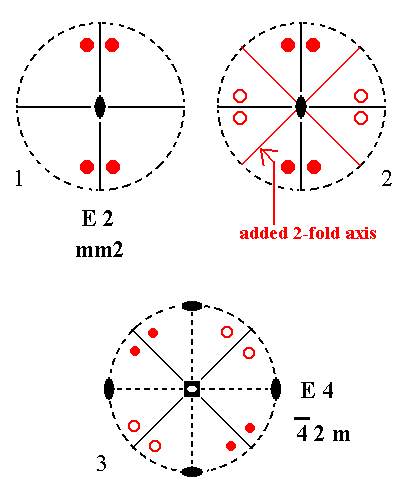Figure 15. E2---E4.
(1). Stereogram of the symmetry elements and of the face poles of the most general Form of the Class mm2 represented by the entry E2.
(2). Addition of a 2-fold rotation axis (red), making angles of 450 with existing mirror planes. The existing mirror planes duplicate this added axis. The motifs (i.e. faces, represented by the projections of face poles) are multiplied by the new 2-fold axes resulting in the motif pattern as indicated.
(3). The newly generated motif pattern turns out to be equivalent to the pattern as it is depicted in the stereogram of the Class E4, so we have generated Class E4 from E2.

```
```
If we add such a 2-fold rotation axis to the symmetry of E2, such that it makes angles of 300 and 600 with the existing mirror planes (while it is still perpendicular to the n-fold axis), then we will obtain the Class G5. See Figure 16.Figure 16. Derivation of G5 from E2.
(1). Stereogram of the symmetry elements, and of the face poles of the most general Form, of the Class
mm2 represented by the entry E2. Two vertical mirror planes perpendicular to each other are present. The (vertical) n-fold axis is here a 2-fold rotation axis.
(2). Addition of a 2-fold rotation axis (indicated by
2) perpendicular to the n-fold axis, making an angle of 300 with an existing mirror plane (indicated by m), and an angle of 600 with the other existing mirror plane.
(3). The first mentioned mirror plane is duplicated by the added 2-fold axis, while also two more motifs are generated (here two lower motifs).
(4). The second mentioned mirror plane (the one that is horizontally drawn in the Figure) reflects that new mirror plane and the new motifs, resulting in yet another mirror plane and two more lower motifs.
(5). The added 2-fold axis is duplicated by the first new mirror plane, resulting in a new 2-fold axis, lying in an existing mirror plane, and as such indicated by
m2 ( So "m2" means mirror plane + 2-fold axis, See arrow).
But because the motifs lying above and below
m2 are now not only related by a reflection, but also by a rotation of 1800, these motifs should have upper counter motifs (because the action of the 2-fold rotation axis generates upper motifs from lower ones). Also the motifs drawn in the upper part of the stereogram should have their lower counter motifs in order to comply with the added 2-fold axis.
(6). The mirror plane, indicated by a green arrow, generates another
m2, indicated by a blue arrow.
(7). The mirror plane, indicated by the blue arrow, duplicates the motifs lying on the right of it, such that we now find such motifs also at the six o'clock position in the stereogram.
One of the originally existing mirror planes, namely the one vertically drawn in the stereograms, produces yet another
m2, indicated by the red arrow, which means that the added 2-fold rotation axis now lies in a mirror plane. This m2 duplicates the motifs as indicated. The resulting motifs are again duplicated by reflection in an already originally existing mirror plane (horizontally drawn in the images). The final result is a motif pattern consisting of six pairs of upper motifs and their corresponding lower motifs.
(8). This motif configuration demands that now all the
m's must be m2's. To understand why this must be, see the Remark (inframe, section Implication of Mirror Planes ) below Figure 1 in Part Four. There we see that after every 300 we must find an m2.
The symmetry configuration so obtained is fully equivalent with that depicted in (9), which is the symmetry configuration of Class G5.
(9). Stereogram of the resulting Class,
6/m 2/m 2/m, represented by the entry G5 of our table of the stereograms of the first 27 Crystal Classes to be derived.

```
```
Addition of a 2-fold rotation axis perpendicular to the n-fold axis of E2 and making angles of 00 and 900 with the existing mirror planes yields G2. See Figure 17.Figure 17. Derivation of G2 from E2 (1). Stereogram of the symmetry elements and of the face poles of the most general Form, of the Class mm2, represented by the entry E2. Two vertical mirror planes and one vertical 2-fold rotation axis are present. (2). Addition of a 2-fold rotation axis perpendicular to the (vertical) n-fold rotation axis and making angles of 00 and 900 with existing mirror planes. (3). The upper motifs must obtain their lower counter motifs in order to comply with the added 2-fold axis. (4). The resulting motif pattern then implies a horizontal mirror plane and one more 2-fold rotation axis, now making up a 2/m 2/m 2/m symmetry.

That a horizontal mirror plane is indeed implied as stated in (4) of Figure 17, is explained in the Remark (inframe) in Part Four, below Figure 1.
From the lower part of Figure 1a of that same Remark we can deduce that also a 2-fold rotation axis is implied.

```
```
Addition of a 2-fold rotation axis perpendicular to the n-fold axis of E3 and making an angle of 450 with an existing 2-fold axis causes a proliferation of 2-fold axes beyond the the number permissible for crystals. See Figure 18.Figure 18. (1). Stereogram of the symmetry elements and of the face poles of the most general Form, of the Class 3*2/m, represented by the entry E3. (2). Addition of a 2-fold rotation axis perpendicular to the (vertical) n-fold axis and making angles of 450 and 150 with existing 2-fold axes. (3). The added 2-fold axis is duplicated by an existing 2-fold axis. The resulting 2-fold axis is then duplicated by an existing mirror plane. The resulting axis is in turn duplicated by an existing 2-fold axis, etc., finally resulting in a forbidden symmetry.

```
```
Addition of a 2-fold rotation axis perpendicular to the (vertical) n-fold axis and making an angle of 300 with existing 2-fold axes, to (the symmetry content of) E3 yields G5. See Figure 19.Figure 19. Derivation of G5 from E3.
(1). Stereogram of the symmetry elements and of the face poles of the most general Form, of the Class
3*2/m, represented by the entry E3.
(2). Addition of a 2-fold rotation axis perpendicular to the (vertical) n-fold axis and making an angle of 300 with existing 2-fold axes.
(3). The added 2-fold axis is multiplied by existing 2-fold axes such that now each vertical mirror plane contains a 2-fold axis (perpendicular to the n-fold axis).
(4). In order to comply with all these horizontal 2-fold rotation axes each existing motif must have its counter motif (An upper one must have its lower, a lower must have its upper counter motif). The resulting motif configuration now demands that all the horizontal 2-fold axes must be contained in vertical mirror planes (See Figure 1e of the Remark (inframe) in Part Four, below Figure1 there. For the implication of a horizontal mirror plane, see that same Remark, Figure 1a.
All this results in a symmetry configuration consistent with that of G5, i.e with
6/m 2/m 2/m symmetry.

Our case of the added 2-fold axis making an angle of 300 with existing 2-fold axes is equivalent to the case where it makes an angle of 900, as can be seen in the stereogram of E3 ((2) in the above Figure).

```
```
We do not have to check the case of the added 2-fold rotation axis making an angle of 600 with existing 2-fold axes, because those existing axes themselves make already an angle of 600 with each other.
```
```
Addition of a 2-fold rotation axis perpendicular to the n-fold axis of E4, and making angles of 300 and 600 with existing 2-fold axes, yields a 12-fold rotational symmetry, forbidden (i.e. impossible) for crystals. See Figure 20.Figure 20. Generation of 12-fold rotational symmetry out of E4.
(1). Stereogram of the symmetry elements and of the face poles of the most general Form of the Class
4*2m represented by the entry E4.
(2). Addition of a 2-fold rotation axis perpendicular to the (vertical) n-fold axis and making angles of 300 and 600 with existing 2-fold rotation axes.
(3). This new axis is then duplicated by one of the existing mirror planes, and the resulting axis is in turn duplicated by one of the existing 2-fold axes. The result is then duplicated by one of the existing mirror planes.
(4). By the action of the (horizontal) 2-fold axes new mirror planes are generated as indicated. The mirror planes are indicated by the symbol
m.
(5). Here -- and already in (4) -- we can see that we have 12 sets of symmetry elements, each set consisting of half a vertical mirror plane
(m) and half a horizontal 2-fold axis
( The latter is indicated by the symbol
2 ). So we have generated a 12-fold rotational symmetry (indicated by 12 sectors, each consisting of a white and a red half sector).
Such a symmetry is -- as we know -- impossible in crystals. Consequently we cannot add a 2-fold rotation axis (perpendicular to the n-fold axis) making angles of 300 and 600 with existing 2-fold axes.

```
```
We do not need to check the effect of an addition of a horizontal 2-fold axis making an angle of 900 with existing 2-fold axes, because the latter are already separated by 900 from each other.

We still must, however, check an angle of 450.
Addition of a 2-fold rotation axis perpendicular to the (vertical) n-fold axis and making an angle of 450 with existing 2-fold axes, to (the symmetry content of) E4 yields G4. See Figure 21.Figure 21. Derivation of G4 from E4.
(1). Stereogram of the symmetry elements and of the face poles of the most general Form of the Class
4*2m represented by the entry E4.
(2). Addition of a 2-fold rotation axis perpendicular to the (vertical) n-fold axis and making an angle of 450 with existing 2-fold axes.
(3). A second 2-fold rotation axis is generated by the existing 2-fold axes. Like the added 2-fold axis the new axis is contained in a vertical mirror plane.
(4). The two 2-fold axes demand that to each motif there must be a counter motif ( To an upper motif a lower one, and to a lower an upper one).
(5). The resulting motif configuration then implies a horizontal mirror plane and two more vertical mirror planes each containing one of the original horizontal 2-fold rotation axes. The resulting symmetry configuration is now exactly equivalent to that of G4.

```
```
Addition of a 2-fold rotation axis perpendicular to the (vertical) n-fold axis and making angles of 300 and (by implication) 900 with existing 2-fold axes, to (the symmetry content of) E5 yields G5. See Figure 22.Figure 22. Derivation of G5 from E5.
(1). Stereogram of the symmetry elements and of the face poles of the most general Form, of the Class
6*m2, represented by the entry E5.
(2). Addition of a 2-fold rotation axis perpendicular to the n-fold axis of the Class E5 and making angles of 300 and 900 with existing 2-fold axes.
(3). The added 2-fold axis generates 12 more motifs as indicated. The resulting motif pattern then implies three more vertical mirror planes (See Figure 1d of the Remark (inframe) in Part Four, below Figure1 there), and three more horizontal 2-fold axes (See Figure 1e of that same Remark, that shows that precisely between two
m2's, separated by 600, always lies another m2.
The resulting symmetry configuration is that of G5.

```
```
We do not have to check the addition of a 2-fold axis making an angle of 600 with an existing 2-fold axis, because such an axis is already present.

When the angle with an existing 2-fold axis would be 450 then also an angle of 150 with an existing 2-fold axis is implied, and we know that such an angle leads to a symmetry configuration that is impossible in crystals.

Summing up : By adding a 2-fold rotation axis perpendicular to the n-fold axis of the Classes of column E yielded the Classes E4, G5, G2 and G4. So nothing new is generated.

```
```
Now we will check the addition of a 2-fold rotation axis perpendicular to the n-fold axis of the Classes of column F.
In adding a 2-fold rotation axis perpendicular to the n-fold axis of F3 (= the first Class of column F) we must check a possible angle of 300 (and by implication 900) with existing mirror planes. It yields the Class E3. See Figure 23.Figure 23. Derivation of E3 from F3.
(1). Stereogram of the symmetry elements and of the face poles of the most general Form, of the Class
3m represented by the entry F3.
(2). Addition of a 2-fold rotation axis perpendicular to the n-fold axis of Class F3 and making an angle of 300 (and by implication an angle of 900) with an existing (vertical) mirror plane.
(3). The added 2-fold axis generates six new motifs as indicated. The resulting motif pattern is then consistent with the symmetry of E3.

```
```
Addition of a 2-fold axis perpendicular to the n-fold axis of F3 and making an angle of 600 with existing mirror planes yields E5. See Figure 24.Figure 24. Derivation of E5 from F3.
(1). Stereogram of the symmetry elements and of the face poles of the most general Form, of the Class
3m represented by the entry F3.
(2). Addition of a 2-fold rotation axis perpendicular to the n-fold axis of F3 and making an angle of 600 with existing mirror planes.
(3). The added 2-fold axis generates six more motifs as indicated. These motifs must be symmetrical with respect to the projection plane (See Figure 1a in the Remark in Part Four, below Figure 1 there).
The resulting motif pattern then demands two more horizontal 2-fold axes (See also Figure 1a of that same Remark).
The resulting symmetry configuration is that of E5.

```
```
An angle of 450 needs not to be checked because it implies an angle of 150 with an existing mirror plane leading to a forbidden symmetry.
```
```
Addition of a 2-fold axis perpendicular to the n-fold axis of F4 and making an angle of 450 with existing mirror planes, yields G4. See Figure 25.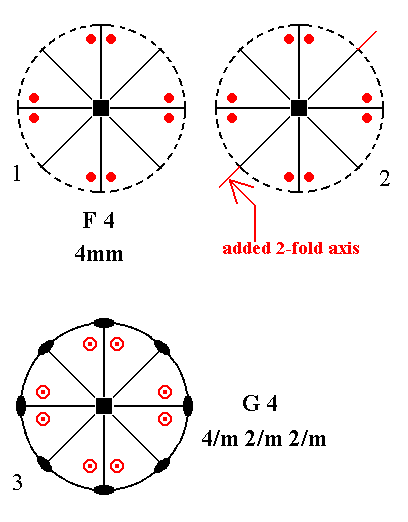Figure 25. Derivation of G4 from F4.
(1). Stereogram of the symmetry elements and of the face poles of the most general Form, of the Class
4mm represented by the entry F4.
(2). Addition of a 2-fold axis perpendicular to the n-fold axis of F4 and making angles of 450 with existing mirror planes.
(3). The added 2-fold axis generates eight more motifs as indicated. The resulting motif pattern then demands a horizontal mirror plane and three more horizontal 2-fold axes. Figure 1a in the Remark (inframe) in Part Four shows that a vertical mirror plane, containing a horizontal 2-fold rotation axis (= the added 2-fold axis), implies the motifs to be symmetric with respect to the plane of projection, so lower counter motifs are generated to comply with that symmetry. These two pairs of motifs are now multiplied four times about the n-fold axis in order to comply with its 4-fold symmetry. The final motif pattern is now generated together with its mentioned implications.
The new symmetry configuration is that of G4.

```
```
An angle of 300 needs not to be investigated because it implies an angle of 150 which leads to a symmetry impossible in crystal. The same applies to an angle of 600.

Addition of a 2-fold rotation axis perpendicular to the n-fold axis of F4 and making an angle of 900 with an existing mirror plane also yields G4. See Figure 26.Figure 26. Derivation of G4 from F4.
(1). Stereogram of the symmetry elements and of the face poles of the most general Form, of the Class
4mm represented by the entry F4.
(2). Addition of a 2-fold axis perpendicular to the n-fold axis of F4 and making an angle of 900 with an existing mirror plane.
(3). The added 2-fold axis generates eight more motifs as indicated. The resulting motif pattern then demands a horizontal mirror plane and three more horizontal 2-fold axes. The new symmetry configuration is that of G4.

```
```
Addition of a 2-fold axis perpendicular to the n-fold axis of F5 yields G5. The added 2-fold axis must be contained in one of the existing mirror planes, otherwise an angle of 150, or smaller, will be involved leading to a forbidden symmetry. See Figure 27.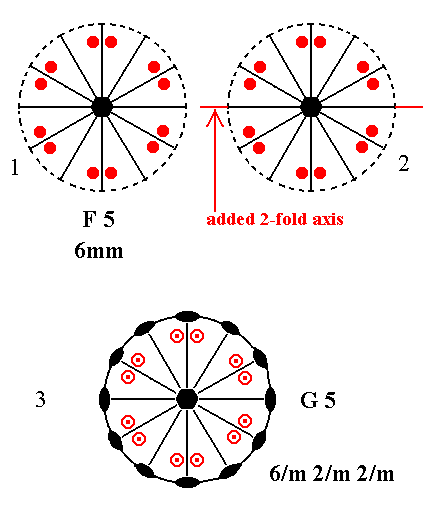Figure 27. Derivation of G5 from F5.
(1). Stereogram of the symmetry elements and of the face poles of the most general Form, of the Class
6mm represented by the entry F5.
(2). Addition of a 2-fold rotation axis perpendicular to the n-fold axis of F5 and contained in one of the existing mirror planes.
(3). The added 2-fold axis generates 12 more motifs as indicated. The resulting motif pattern demands a horizontal mirror plane and 5 more horizontal 2-fold rotation axes. The symmetry configuration is now that of G5.

```
```
Summing up : Addition of a 2-fold rotation axis perpendicular to the (vertical) n-fold axis of the Classes of column F yields the following Classes : E3, E5, G4 and G5.
So nothing new is generated.
```
```
Finally, we will investigate the effect of the addition of a 2-fold rotation axis perpendicular to the (vertical) n-fold axis of the Classes of column G.
Addition of a 2-fold rotation axis perpendicular to the n-fold axis of G2 (= the first Class of column G) and making angles of 300 and 600 with existing 2-fold axes, yields G5.
See Figure 28.Figure 28. Derivation of G5 from G2.
(1). Stereogram of the symmetry elements and of the face poles of the most general Form, of the Class
2/m 2/m 2/m represented by the entry G2.
(2) Addition of a 2-fold rotation axis perpendicular to the n-fold axis of G2 and making angles of 300 and 600 with existing horizontal 2-fold axes.
b duplicates the existing axis a resulting in the 2-fold axis c. The existing axis d then generates axis e. The existing axis d is then duplicated by axis e resulting in axis f. We now have six horizontal 2-fold rotation axes. In virtue of these axes 16 more motifs are generated, ending up with a motif configuration as depicted. The symmetry configuration is now that of G5 ( In the stereogram in (4) the motifs are drawn a little bit reshuffled, but this is immaterial with respect to symmetry, because their position is still general).

```
```
Addition of a 2-fold rotation axis perpendicular to the n-fold axis of G2 and making angles of 450 with existing 2-fold axes yields G4. See Figure 29.Figure 29. Derivation of G4 from G2.
(1). Stereogram of the symmetry elements and of the face poles of the most general Form, of the Class
2/m 2/m 2/m represented by the entry G2.
(2). Addition of a 2-fold rotation axis perpendicular to the n-fold axis of G2 and making angles of 450 with existing horizontal 2-fold axes.
(3). The added 2-fold axis generates eight more motifs as indicated. The resulting motif pattern demands two more vertical mirror planes and one more horizontal 2-fold axis. It also demands that the (vertical) n-fold axis, which was originally a 2-fold axis, becomes a 4-fold rotation axis. The resulting symmetry configuration is now that of G4.

```
```
We do not have to investigate an angle of 900 because existing 2-fold axes already make such an angle with each other.
```
```
Addition of a 2-fold rotation axis perpendicular to the n-fold axis of G4 (= the second Class of column G) does not make any difference :
If the angle of this added 2-fold axis is 300 or 600 then an angle of 150 will be involved, leading to a forbidden symmetry.
With respect to 450 and 900 :   Such 2-fold axes are already present. See Figure 30.Figure 30. G4---G4.

```
```
The same applies to the addition of a 2-fold rotation axis perpendicular to the n-fold axis of G5 :
2-fold axes making angles of 300, 600 and 900 with other 2-fold axes are already present.
If we would add a 2-fold axis (perpendicular to the n-fold axis) making an angle of 450 then an angle of 150 will be involved leading to a forbidden symmetry. See Figure 31.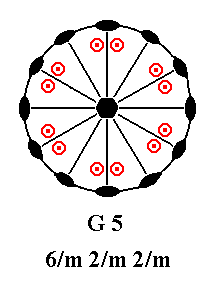Figure 31. G5---G5.

```
```
Summing up : Addition of a 2-fold rotation axis perpendicular to the (vertical) n-fold axis of the Classes of column G generated the following Classes : G5 and G4. So nothing new is actually generated.
```
```
In the next Part we will continue our investigation with respect to the question whether any new Classes will be generated (new with respect to the already derived Classes each of which possessing at most one more-than-2-fold axis) when the above mentioned symmetry elements (a center of symmetry, a 2-fold rotation axis perpendicular to the n-fold axis, a mirror plane parallel to the n-fold axis and a mirror plane perpendicular to that axis) are added to the (symmetry content of the) Classes of the columns D, E, F and G. We have done this already with respect to (the addition of) a center of symmetry and with respect to (the addition of) a 2-fold rotation axis perpendicular to the (vertical) n-fold axis (of the Classes of these columns).
No new Classes were found so far.
```

```
```